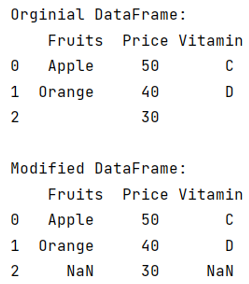# How to replace blank values (white space) with NaN in Pandas?

Given a Pandas DataFrame, we have to replace blank values (white space) with NaN. By Pranit Sharma Last updated : September 22, 2023

Pandas is a special tool that allows us to perform complex manipulations of data effectively and efficiently. Inside pandas, we mostly deal with a dataset in the form of DataFrame. DataFrames are 2-dimensional data structures in pandas. DataFrames consist of rows, columns, and the data.

While creating a DataFrame or importing a CSV file, there could be some NaN values in the cells. NaN values mean "Not a Number" which generally means that there are some missing values in the cell.

## Problem statement

Given a Pandas DataFrame, we have to replace blank values (white space) with NaN.

## Replacing blank values with NaN in Pandas

For this purpose, we are going to use DataFrame.replace() method. This method is used to replace a string, regex, list, dictionary, series, number, etc. The syntax of this method is:

```DataFrame.replace(
to_replace=None,
value=_NoDefault.no_default,
*,
inplace=False,
limit=None,
regex=False,
method=_NoDefault.no_default
)
```
Note

To work with pandas, we need to import pandas package first, below is the syntax:

```import pandas as pd
```

Let us understand with the help of an example,

## Python program to replace blank values with NaN in Pandas

```# Importing pandas package
import pandas as pd

# Imorting numpy package
import numpy as np

# Creating dictionary
d = {
'Fruits':['Apple','Orange',' '],
'Price':[50,40,30],
'Vitamin':['C','D',' ']
}

# Creating DataFrame
df = pd.DataFrame(d)

# Display original DataFrame
print("Orginial DataFrame:\n",df,"\n")

# Replacing blank values with NaN values
df = df.replace(' ', np.nan, regex=True)

# Display modified DataFrame
print("Modified DataFrame:\n",df)
```

### Output

The output of the above program is: